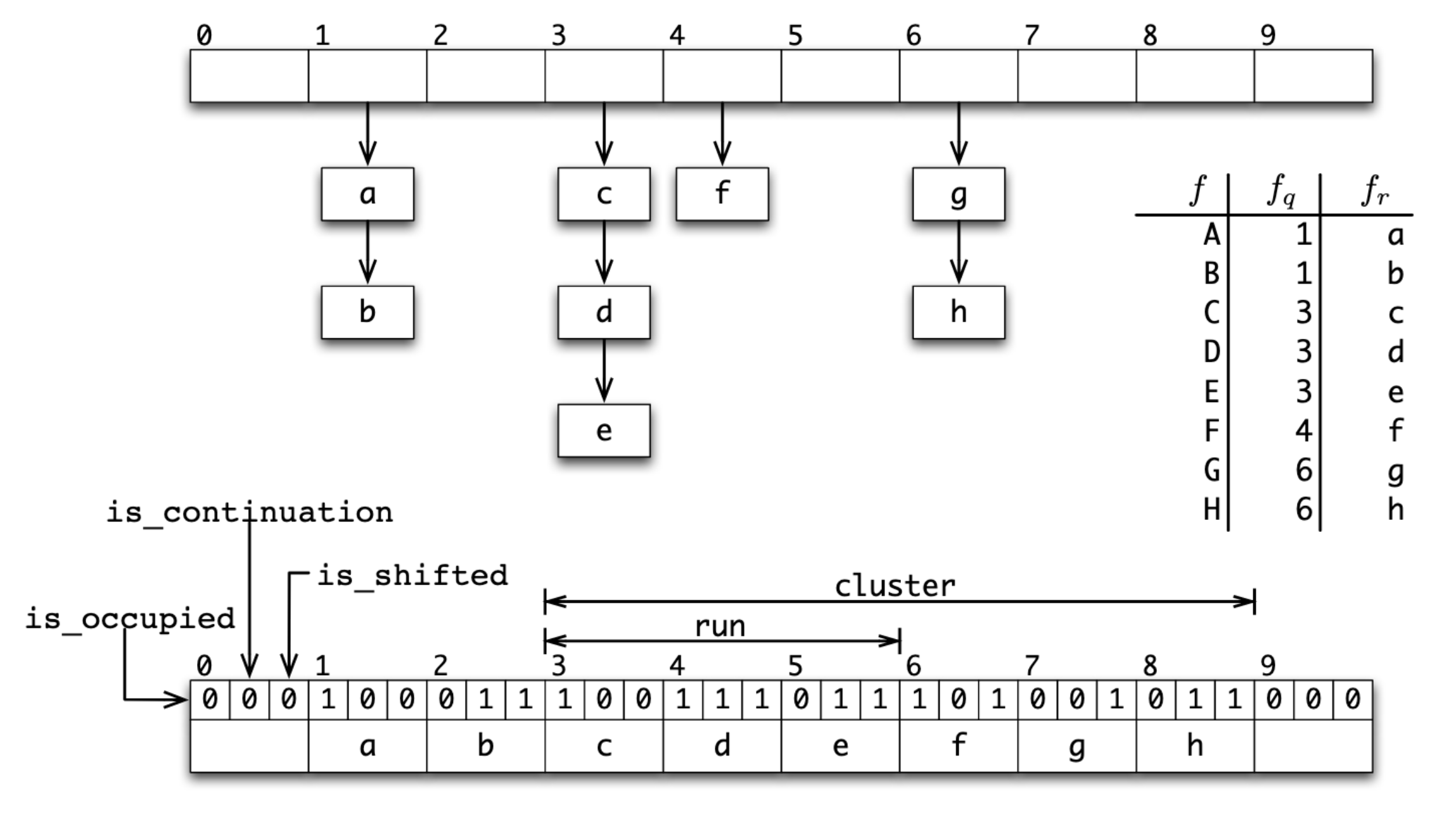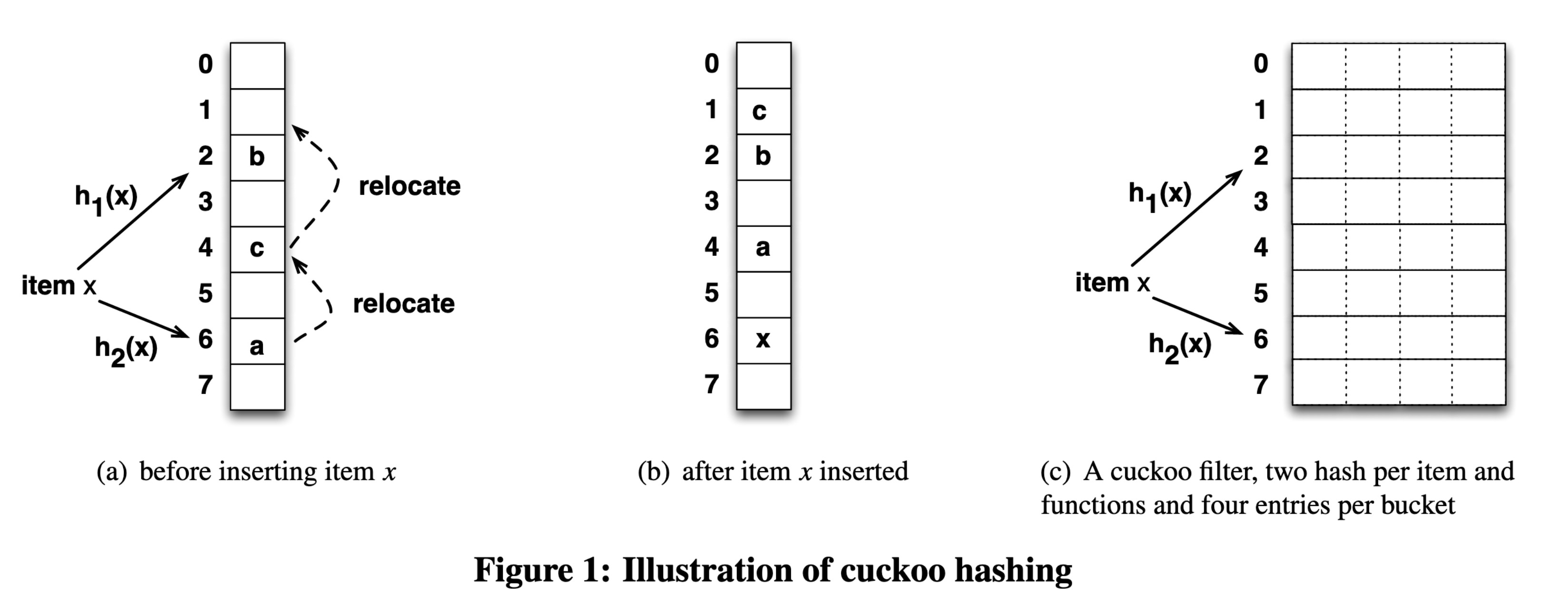Bloom filter 是一種機率資料結構（probabilistic data structure），類似於集合，常用於需快速驗證成員是否「可能存在」或是「絕對不存在」在容器中，亦即有機會出現假陽性（false positive），但絕不會有假陰性（false negative）。

Bloom filter 的優勢是：

Bloom filter 則有以下短處：

• 經典款 Bloom filter 容器大小固定（fixed-size），無法動態調整儲存空間。
• 可能給出假陽性答案：回報存在但實際不存在，且錯誤隨數量變多上升。
• 自身不儲存成員資料，需要有額外的儲存資料方案。
• 只能新增成員，但不能移除成員（可透過變形解決）。
• 若輸入資料集本身離散，接近隨機存取，無法充分利用 CPU cache。
• 承上，因為隨機存取，不利於延伸到記憶體以外的外部儲存裝置。

Bloom filter 常見應用場景為：

## 概念#

Bloom filter 由下列兩個部分組成：

• 一個 $m$ 位元的位元陣列（bit array）
• $k$ 個不同的雜湊函數

• 新增： 新增一個值時，透過 $k$ 個雜湊函數產生 $k$ 個雜湊值，分別代表在位元陣列的索引位置，再將 $k$ 個位置的位元翻轉至 1。
• 查詢： 同樣透過 $k$ 個雜湊函數產生 $k$ 個雜湊值作為位元陣列的索引位置，若所有位元皆為 1，則代表該值存在。• 欲儲存一百萬個元素，需要多少位元？
• 出現假陽性的機率是多少？可以調整嗎？
• 需要幾個雜湊函數？
• 可重複使用相同的雜湊函數嗎？

1. 預期會儲存多少 $n$ 個元素到容器。
2. 可容忍的假陽性機率 $\epsilon$，即容器不包含該元素，檢測卻回報存在（所有雜湊位皆為 1）。

$$m = -\frac{n \ln{\epsilon}}{(\ln{2})^2}$$

$$k = -\frac{\ln{\epsilon}}{\ln{2}} = -\log_2{\epsilon}$$

$$g_i(x) = h_1(x) + ih_2(x)$$

## 架構設計#

### 利用 Vec 儲存位元#

Bloom filter 底層以位元陣列作為儲存容器，如果目標是最省空間，該用 Rust 的什麼型別來儲存位元呢？

pub struct BloomFilter {
bits: [bool; N]
}


pub struct BloomFilter {
bits: [bool]
}


fn main() {
let input_len = 5;
let bits = [true; input_len];
BloomFilter { bits };
}


error[E0435]: attempt to use a non-constant value in a constant
--> src/main.rs:7:23
|
7 |     let bits = [true; input_len];
|                       ^^^^^^^^^ non-constant value

error[E0277]: the size for values of type [bool] cannot be known at compilation time
--> src/main.rs:8:5
|
8 |     BloomFilter { bits };
|     ^^^^^^^^^^^^^^^^^^^^ doesn't have a size known at compile-time


pub struct BloomFilter {
bits: Vec<bool>
}


### 儲存兩個雜湊函數#

use std::collections::hash_map::DefaultHasher;

pub struct BloomFilter {
/// The bit array of _m_ bits.
bits: Vec<bool>,
/// Count of hash functions. Denoted by _k_.
hash_fn_count: usize,
/// The hashers that do real works.
hashers: [DefaultHasher; 2],
}


### 使用 PhantomData 讓編譯器閉嘴#

1. 泛型型別參數宣告跟著 struct： 也就是 struct BloomFilter<T>，如此一來，一個容器實例只能操作一種型別，而且在編譯期就決定。
let bf = BloomFilter::new();
bf.insert(&1);            // infer the type T is i32
bf.insert("wront type");  // compile error: &str is not compatible to i32

2. 泛型型別跟著 struct 的方法，struct 本身不宣告： 很難想像容器裡面儲存不同的型別，但 BloomFilter 實際上只需要一個 Vec<bool> 記錄存在與否，到底新增了什麼型別的元素其實不重要，型別有實作雜湊就行。這個作法下，你可能會看到這種邪魔歪道：
let bf = BloomFilter::new();
bf.insert(&1);
bf.insert("another type");  // it works
bf.insert(vec![3.14159]);   // it also works


use std::collections::hash_map::DefaultHasher;

- pub struct BloomFilter {
+ pub struct BloomFilter<T> {
/// .. snip
}


error[E0392]: parameter T is never used
--> src/lib.rs:3:24
|
3 | pub struct BloomFilter<T> {
|                        ^ unused parameter
|
= help: consider removing T, referring to it in a field, or using a marker such as std::marker::PhantomData


use std::collections::hash_map::DefaultHasher;
use std::marker::PhantomData;

pub struct BloomFilter<T> {
/// The bit array of _m_ bits.
bits: Vec<bool>,
/// Count of hash functions. Denoted by _k_.
hash_fn_count: usize,
/// The hashers that do real works.
hashers: [DefaultHasher; 2],
_phantom: PhantomData<T>,
}


## ?Sized 讓容器可以儲存 DSTs#

use std::collections::hash_map::DefaultHasher;
use std::marker::PhantomData;

- pub struct BloomFilter<T> {
+ pub struct BloomFilter<T: ?Sized> {
// .. snip
}


## 基本操作#

Bloom filter 為類似集合的容器，當然有類似的操作，事實上，這類機率性集合成員檢測的資料結構有個較少聽見但令人印象深刻的名字，稱為「Approximate Membership Query（AMQ）」，提供 add(element)query(element) 兩個基本操作。

• new：初始化一個容器。
• insert：新增一個元素。
• contains：檢查容器內有無特定元素（是否曾新增過）。

• make_hash：給定輸入元素資料，透過兩個雜湊函數產生兩個雜湊值。
• get_index：將 make_hash 的兩雜湊值帶入 $g_i(x) = h_1(x) + ih_2(x)$ 計算單次 i 的索引位置。
• optimal_bits_count：給定預期儲存元素個數 $n$ 與假陽性機率 $\epsilon$，得位元陣列最適位元數 $m$。
• optimal_hashers_count：給定預期假陽性機率，得最適雜湊函數個數 $k$。

### 初始化#

Bloom filter 有四個參數 $m$、$n$、$k$、$\epsilon$ 可以調整（詳見 效能一節），對使用者來說，有幾個雜湊函數或底層是多少個位元都是實作細節了，更關心的可能是

「我有一百萬筆資料需要驗證存在，容錯率需要在 0.1%，我需要多大的儲存空間？」

pub fn new(capacity: usize, err_rate: f64) -> Self;


/// m = -1 * (n * ln ε) / (ln 2)^2
fn optimal_bits_count(capacity: usize, err_rate: f64) -> usize {
let ln_2_2 = std::f64::consts::LN_2.powf(2f64);
(-1f64 * capacity as f64 * err_rate.ln() / ln_2_2).ceil() as usize
}

/// k = -log_2 ε
fn optimal_hashers_count(err_rate: f64) -> usize {
(-1f64 * err_rate.log2()).ceil() as usize
}


pub fn new(capacity: usize, err_rate: f64) -> Self {
let bits_count = Self::optimal_bits_count(capacity, err_rate);
let hash_fn_count = Self::optimal_hashers_count(err_rate);
}


pub fn new(capacity: usize, err_rate: f64) -> Self {
// #1 Get optimal count of bit
let bits_count = Self::optimal_bits_count(capacity, err_rate);
// #2 Get optimal count of hash functions
let hash_fn_count = Self::optimal_hashers_count(err_rate);
// #3 Use RandomState to build different hasher
let hashers = [
RandomState::new().build_hasher(),
RandomState::new().build_hasher(),
];

Self {
bits: vec![false; bits_count], // #4 Initialize a all zero bit array
hash_fn_count,
hashers,
_phantom: PhantomData,
}
}

1. 透過指定假陽性機率與預期元素個數，算得最適位元數
2. 透過指定假陽性機率，算得最適雜湊函數個數
3. 透過 std 內建的 RandomState 產生兩個不同初始狀態的雜湊函數，以模擬 $k$ 個雜湊函數
4. 初始化一個全零的位元陣列

### 新增#

pub fn insert(&mut self, elem: &T)
where
T: Hash,
{
let hashes = self.make_hash(elem);  // #1
for fn_i in 0..self.hash_fn_count { // #2
let index = self.get_index(hashes, fn_i as u64); // #3
self.bits[index] = true;        // #4
}
}

1. 取得 $h_1(x)$ 和 $h_2(x)$ 的雜湊輸出結果。
2. 迭代 i 次，i 上限為 $k$：初始化時所得的最適雜湊函數個數
3. 計算 $g_i(x) = h_1(x) + ih_2(x)$ 取得索引位置
4. 將索引位置下的位元設定為 1

fn make_hash(&self, elem: &T) -> (u64, u64)
where
T: Hash,
{
// #1
let hasher1 = &mut self.hashers.clone();
let hasher2 = &mut self.hashers.clone();

// #2
elem.hash(hasher1);
elem.hash(hasher2);

// #3
(hasher1.finish(), hasher2.finish())
}

1. 為保存兩個 hasher 內部初始狀態，使用 clone 複製新的 hasher 來做雜湊
2. elem 餵給 hasher 計算雜湊值
3. 輸出雜湊值，由於 Hasher::finish 不會重設 hasher 內部狀態，所以需要步驟一 clone 來保留 hasher 的原始狀態

fn get_index(&self, (h1, h2): (u64, u64), fn_i: u64) -> usize {
(h1.wrapping_add(fn_i.wrapping_mul(h2)) % self.bits.len() as u64) as usize
}


### 查詢#

pub fn contains(&self, elem: &T) -> bool
where
T: Hash,
{
let hashes = self.make_hash(elem); // #1
(0..self.hash_fn_count).all(|fn_i| { // #1 使用 iter
let index = self.get_index(hashes, fn_i as u64);
self.bits[index]
})
}

1. 取得 $h_1(x)$ 和 $h_2(x)$ 的雜湊輸出結果。
2. 使用 Iterator::all 迭代收集 $k$ 個雜湊函數的輸出
3. 計算 $g_i(x) = h_1(x) + ih_2(x)$ 取得索引位置
4. 回傳索引位置下的位元 bool 值，此值會匯集起來，於步驟二確認命中全部 $k$ 個索引，即為元素存在

pub struct BloomFilter<T: ?Sized> {
bits: Vec<bool>,
hash_fn_count: usize,
hashers: [DefaultHasher; 2],
_phantom: PhantomData<T>,
}

impl<T: ?Sized> BloomFilter<T> {
pub fn new(capacity: usize, err_rate: f64) -> Self {
let bits_count = Self::optimal_bits_count(capacity, err_rate);
let hash_fn_count = Self::optimal_hashers_count(err_rate);
let hashers = [
RandomState::new().build_hasher(),
RandomState::new().build_hasher(),
];

Self {
bits: vec![false; bits_count],
hash_fn_count,
hashers,
_phantom: PhantomData,
}
}

pub fn insert(&mut self, elem: &T)
where
T: Hash,
{
// g_i(x) = h1(x) + i * h2(x)
let hashes = self.make_hash(elem);
for fn_i in 0..self.hash_fn_count {
let index = self.get_index(hashes, fn_i as u64);
self.bits[index] = true;
}
}

pub fn contains(&self, elem: &T) -> bool
where
T: Hash,
{
let hashes = self.make_hash(elem);
(0..self.hash_fn_count).all(|fn_i| {
let index = self.get_index(hashes, fn_i as u64);
self.bits[index]
})
}

fn get_index(&self, (h1, h2): (u64, u64), fn_i: u64) -> usize {
(h1.wrapping_add(fn_i.wrapping_mul(h2)) % self.bits.len() as u64) as usize
}

fn make_hash(&self, elem: &T) -> (u64, u64)
where
T: Hash,
{
let hasher1 = &mut self.hashers.clone();
let hasher2 = &mut self.hashers.clone();

elem.hash(hasher1);
elem.hash(hasher2);

(hasher1.finish(), hasher2.finish())
}

/// m = -1 * (n * ln ε) / (ln 2)^2
fn optimal_bits_count(capacity: usize, err_rate: f64) -> usize {
let ln_2_2 = std::f64::consts::LN_2.powf(2f64);
(-1f64 * capacity as f64 * err_rate.ln() / ln_2_2).ceil() as usize
}

/// k = -log_2 ε
fn optimal_hashers_count(err_rate: f64) -> usize {
(-1f64 * err_rate.log2()).ceil() as usize
}
}


## 效能#

Notation Description
$n$ 預期儲存 $n$ 個元素到容器中
$m$ 使用 $m$ 位元的位元陣列來儲存
$k$ 有 $k$ 個雜湊函數計算索引位置
$\epsilon$ 假陽性錯誤的機率 $\epsilon$

Operation Time complexity
insert(v) $O(k)$
contains(v) $O(k)$

## 變形#

• Data locality 不夠好：Bloom filter 底層儲存是位元陣列隨機存取，較不符合現代 CPU 架構的 cache line 使用姿勢。Cloudflare 技術部落格文 When Bloom filters don’t bloom 以幽默筆法帶出這個問題，值得一讀。
• 雜湊次數過多：Bloom filter 每一個新增查詢操作都需要雜湊 $k$ 次，就算利用 double hashing 還是要雜湊兩次，比起其他類似資料結構硬生生多雜湊數次。
• 位元陣列大小固定：Bloom filter 容器大小固定，給你預期的元素個數後，無法動態調整儲存空間，
• bits per entry 較高：以類似功能的的資料結構來說，Bloom filter 在空間利用率上，欲維持一定的假陽性機率，每個元素所需位元數相對較高，需要 $1.44 \log_2{\frac{1}{\epsilon}}$ 個位元。

📚 維基百科

### 動態適應空間大小的 Scalable Bloom Filter#

📚 論文連結

Scalable Bloom Filter 的特色是：動態適應空間大小，不需事先知道預期儲存的元素個數。

Scalable Bloom Filter 的實作蠻暴力的，本身是由一至多個經典款 Bloom filter 組成，若一個 filter 滿了（超過 fill ratio），則會新增一個 filter，往後所有新增都在這個新 filter 上面，直到它也滿了，可視為一個 recursive data structure。

• 成長因子 $s$：每個新增的 filter 空間大小成長率，論文的經驗法則得出預期小成長趨勢選擇 $s = 2$，有較大成長趨勢則 $s = 4$ 效果好。
• 錯誤緊縮率 $r$： 每個新增的 filter 會以等比級數得到更緊縮的假陽性機率上限，由於是等比級數，逼近極限時會小於原始機率，這讓整體假陽性機率得以保持。論文中實證 0.8 到 0.9 在元素預期有大成長率下有最佳平均空間利用率。

### Quotient filter#

📚 論文連結（直接讀論文更易懂）

• 使用 linear probing 解決雜湊碰撞，data locality 好，有 cache friendly。
• 有額外儲存資訊，可在不重建不 rehash filter 的情況下支援刪除、合併、調整空間。
• 綜合上述兩點，非常適合 LSM-tree 等需要存取 SSD 的場景，大幅減少 I/O。
• Throughput 受到雜湊表 load factor 影響較大。
• 空間用量仍比經典款 Bloom filter 多 10% 到 25%。Quotient filter 與它等價的 open addressing hash map

Image Source: Bender, et al., 2012. “Don’t Thrash: How to Cache Your Hash on Flash”.

### 支援刪除元素的 Cuckoo filter#

📚 論文連結（有趣易讀，誠摯推薦）

Cuckoo hashing 是一種解決雜湊碰撞的方法，透過一次計算兩個雜湊函數產生兩個索引位置，若其中一個位置有空位則插入空位，若都沒有空位，則隨機踢掉一個，被踢掉的再去找下一個替死鬼，直到全部都有位置，或踢掉次數大於一定值則停止。這種行為和杜鵑鳥（cuckoo、布穀鳥）鳩佔鵲巢的生物習性很像，因此得名。

Cuckoo filter 利用雜湊表為底層儲存容器，來做集合成員檢測的 AMQ，會和 cuckoo 扯上關係則是因為使用 Cuckoo hashing 解決雜湊碰撞，以增加空間使用率（達到 95% occupancy）。Cuckoo filter 的雜湊表和 Quotient filter 一樣，為了減少空間使用量而只儲存 partial-key。

$$h_1(x) = hash(x) \\ h_2(x) = h_1(x) \oplus hash(fingerprint(x))$$

$$j = i \oplus hash(fingerprint(x))$$

Cuckoo filter 的特性是：

• 支援動態新增與刪除元數。
• 比其他 filter 變形（例如 Quotient filter）好實作，如果懂 Cuckoo hashing 的話。
• 查詢效能比經典款 Bloom filter 好，bits per item 也比較低（$(\log_2{\frac{1}{\epsilon}} + 2) / \alpha$，$\alpha$ 是雜湊表的 load factor，通常為 95.5%）。
• 缺點是「一定要先新增過一個元素，才能對 filter 刪除該元素」，但這是所有支援刪除的 filter 的通病，不然就會有假陽性發生。Image Source: Fan, et al., 2014. “Cuckoo Filter: Practically Better Than Bloom”.